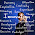Sunday, September 16, 2012

Logical Operators (Bitwise and Boolean) in C#

C# provides many operators which are widely used in various applications depending upon their use. This operators are very basic and everyone knows about it but few thing i wanted to highlight in this post which might not known to many developers. So In this post i will explain few important things about Logical and Bitwise AND/OR/XOR operators with example.

Logical AND (&&) Operator

Logical AND performs Boolean operation with two expression and returns true if both expressions are true and returns false if any one expression is false. If first expression is false then it will not check another expression and returns falseLet’s have a look on below code snippet.

int x = 3;
int y = 0;

if ((y > x) && (x / y > 0))
Console.WriteLine("Logical AND Operation");

The above code will run and compile successfully. In above code second expression seems to throw Dividebyzero exception but first expression returns false so it will not evaluate second expression and assume that first expression is false so entire expression is false.

Logical OR (||) Operator

If we perform logical OR in above example, if first expression is true then it will not evaluate second expression. In logical OR any one expression must be true if first expression is true then others will be skipped.

Note - The disadvantage of logical operators are if second expression doesn’t evaluate then there might be possibility for bug in application. These types of bugs are difficult to find. Sometimes logical operators are called as short circuit operators as well.

Bitwise AND (&) operator

Bitwise operator can operate on integral and Boolean expression. They can operate with two operands and both operands must be evaluated. If first expression returns false even though it will evaluates second expression. Let's have a look on below code.

int x = 3;
int y = 0;

if ((y > x) & (x / y > 0))
Console.WriteLine("Bitwise AND Operation");

The above code will throw an exception because bitwise (&) AND evaluates both expressions and as per above code second expression throws dividebyzero exception. This above example shows bitwise Boolean condition. There are four possible values for Boolean bitwise AND (&) operations.

 Bitwise AND (&) Expression 1 Expression 2 Result True True True True False False False True False False False False

Similarly we can perform bitwise AND on integral numbers as well. This bitwise AND on integral numbers will first convert numbers into binary and then perform AND operations on it. Let’s have a look on below code.

int x = 5;  // Binary Equivalent  - 0101
int y = 7;  // Binary Equivalent  - 0111

//   0101
// & 0111
//  -----
//   0101

int result = x & y; //output  = 5

The above code demonstrates bitwise AND (&) operation between variable x and y. The binary equivalent of x is 0101 and y is 0111. Now bitwise AND (&) operation will perform AND between binary values of x and y as displayed in commented code above. The result will return 5 in decimal number.

Bitwise OR (|) operator

Similarly we can perform Bitwise OR between two operands like bitwise AND. Bitwise OR performs OR operation on binary equivalent numbers. Let’s have look on below code which is modified version of above code.

int x = 5;  // Binary Equivalent  - 0101
int y = 7;  // Binary Equivalent  - 0111

//   0101
// | 0111
//  -----
//   0111

int result = x | y; //output  = 7

In above code Bitwise OR will be applied to the binary equivalent numbers of x and y values. Below is the table which explains bitwise OR results between two expressions.

 Bitwise OR (|) Expression 1 Expression 2 Result True True True True False True False True True False False False

Bitwise Exclusive OR (^) operator

Bitwise Exclusive OR can be applied to two operands and behave similar to Bitwise AND/OR. This performs XOR operation between binary equivalent numbers. Below table explains bitwise XOR results between two expressions.

 Bitwise XOR Expression 1 Expression 2 Result True True False True False True False True True False False False

Usage of Bitwise Operators

Bitwise operators are used in multiple places and also called as fundamental operators on computers. Below are few areas where bitwise operators majorly used.
• Low level programming
• Hardware device drivers creation
• Creating protocols
• Communicating with ports and sockets
• Graphics driver
• Gaming
• Communicating with physical devices
• Encryption
• Data Compression

Hope this post helps you to understand basic concept of Logical and Bitwise Operators in Dotnet. Please leave your feedback in comments section below.

1 comment:

1.